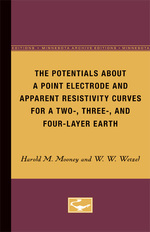# The Potentials About a Point Electrode and Apparent Resistivity Curves for a Two-, Three-, and Four-Layer Earth

HAROLD M. MOONEY
W. W. WETZEL
Edition: NED - New edition
Pages: 158
https://www.jstor.org/stable/10.5749/j.ctttthrc

Export Selected Citations Export to NoodleTools Export to RefWorks Export to EasyBib Export a RIS file (For EndNote, ProCite, Reference Manager, Zotero, Mendeley...) Export a Text file (For BibTex)
1. Front Matter
(pp. [i]-[ii])
2. Acknowledgments
(pp. [iii]-[iv])
Harold M. Mooney and W. W. Wetzel
(pp. [v]-2)
4. Summary
(pp. 3-3)
5. I Use of the Tables and Curves
(pp. 4-13)

It is sometimes important to determine the geologic structure of the earth to depths of a few tens or hundreds of feet at a particular location. Many engineering fields require information on depth to bedrock, to sand, gravel, or clay layers, or to consolidated materials. Some examples of applications are mining operations, highway construction, damsite location, water supply problems, and geologic structure studies.

The electrical resistivity method is one of two geophysical techniques that can sometimes provide this information without drilling. In practice the geophysical methods do not replace drilling, but may greatly reduce the amount needed. Drilling is often...

6. II Mathematical Basis for the Tables and Curves
(pp. 14-24)

Physical statement: Electric current flows into the earth from a point source on the surface. The earth within the volume of interest consists of four horizontal layers. Compute the electric potential at a point on the surface. (See Figure 3.)

Mathematical statement: A point source of current lies in the horizontal plane z =0. The material above this plane has infinite resistivity. Below the plane, discontinuities in electrical resistivity exist at depths z =D1,D2,D3, and infinity. The corresponding resistivities areR1,R2,R3, andR4. Compute a solution of Laplace’s equation at the point (r,θ,...

7. III Tables
(pp. 25-145)

The values ofC1,C2,C3, etc. (see Section H of Part II) are tabulated for various values ofrandt. These coefficients permit numerical evaluation of the fundamental integral of equation 5. They can be applied to computing potentials and apparent resistivities foranynumber of horizontal layers, ofanythickness and resistivity, usinganyelectrode arrangement. The necessary preliminary to their use is the calculation ofA(t) from equation 2.

The accuracy of the computed potentials depends upon the behavior ofA(t). We have assumed thatA(t) can be adequately approximated, over small intervals oft, by...

8. Bibliography
(pp. 146-146)